Back to index
RootMinMax for the HP-42S

By Namir Shammas

RootMinMax is a program that finds the roots or the optimum points of a function. The program uses a straightforward version of Newton's algorithm to find the root of a function or it's first derivative.

x' = x - f(x) / f'(x)

To find the root, and,

x' = x - f'(x) / f''(x)

To find a minima or maxima. The program uses the following approximation to calculate the first and second derivatives:

f'(x) = (f(x+h) - f(x-h)) / 2h  (to calculate the maxima/minima)
= (f(x+h) - f(x)) / h     (to calculate the root)
f''(x) = (f(x+h) - 2f(x) + f(x-h) / h^2

where h = 0.01 * (1 + Abs(x))

Usage:
1. To start the program execute XEQ "RTMIN"
2. The program prompts you for the name of the function in Alpha mode.
3. To find the root, execute XEQ B and respond to the prompts for the guess, maximum number
4. of iterations, and tolerance. The program will iterate and display the root, function value at the root, and the number of iterations.
5. To find the minimum or maximum, execute XEQ C and respond to the prompts for the guess, maximum number of iterations, and tolerance. The program will iterate and display the min/max value, function value, slope value, and second derivative at the located value, and the number of iterations. When the second derivative is positive, the point is a minimum. By contrast, when the second derivative is positive, the point is a maximum.
6. To select a new function execute XEQ A and enter the new function name.
Note: To use previous values for the guess, maximum number of iterations, and tolerance, simply press the R/S key.

Example:

The label AA implements the following sample function:

f(x) = exp(x) - 3 * x^2

Given f(x) find the following:

1. The root new x = 5.
2. The minima/maxima near x = 4
Allow 55 iterations at most and use a tolerance of 1E-7.

XEQ "RTMIN"
FX NAME?
AA
RUN
XEQ B
ROOT GUESS?
5.00000    RUN
MAX ITER?
55.00000    RUN
TOLR?
1E-7    RUN
R01=             5.00000
R01=             4.40206
R01=             3.99213
R01=             3.78989
R01=             3.73813
R01=             3.73332
R01=             3.73309
R01=             3.73308
R01=             3.73308
ROOT=3.73308
RUN
FX=3.57079E-7
RUN
ITER=9.00000
RUN
XEQ C
MIN MAX?
4.00000    RUN
MAX ITER?
55.00000    RUN
TOLR?
1E-7    RUN
R01=             4.00000
R01=             3.37006
R01=             2.98586
R01=             2.84870
R01=             2.83296
R01=             2.83277
MIN%MAX=2.83277
RUN
FX=-7.08129
RUN
SLOPE=-4.98498E-7
RUN
2ND DERIV=10.99454
RUN
ITERS=6.00000
RUN
The program found a root at x = 3.73308 and a min/max point at x = 2.83277. Since the second derivative is positive, the program found a minimum at x = 2.83277

Technical notes:

Memory usage

R00 = Function name
R01 = x
R02 = h
R03 = f(x)
R04 = f(x+h)
R05 = f(x-h)
R06 = Max Iters
R07 = Iters
R08 = Tolerance

Flag 00 is used and is cleared when the program ends.

Binary files for emulators:
Raw binary: RootMinMax.raw  Binary for HP-42X: RootMinMax.42x (HP-48) RootMinMax49.42x (HP-49)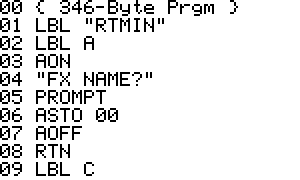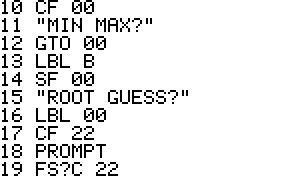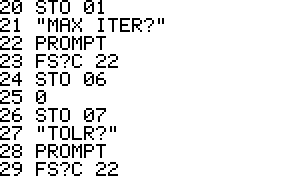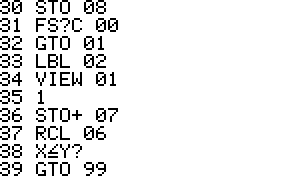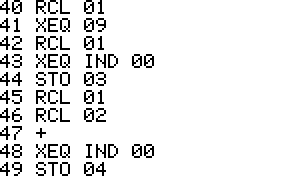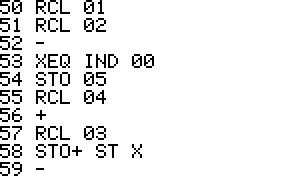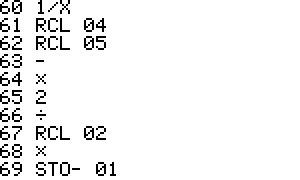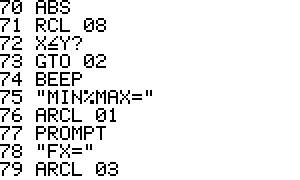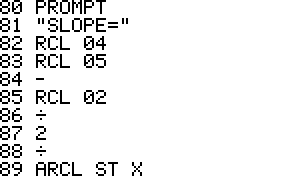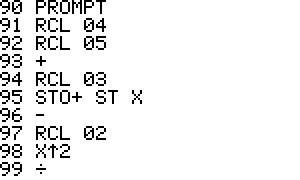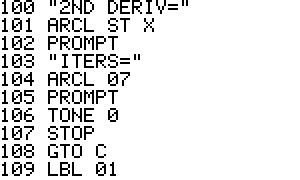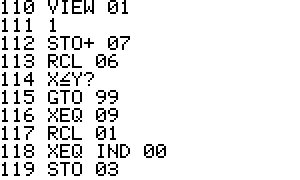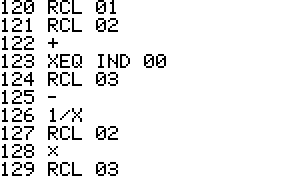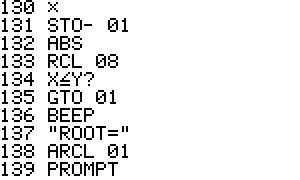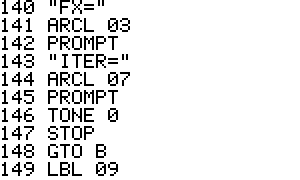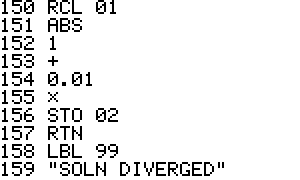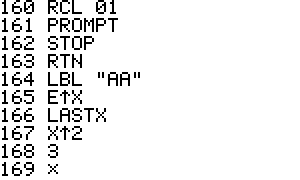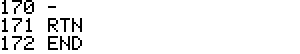Generated by 42s2html - 27 April 2005, 22:49